Guide to Vector Version (sspropv, sspropvc)

Background

The vector version of the SSPROP solves the coupled nonlinear Schrödinger equations for propagation in a birefringent fiber. The code can model birefringence, differential group delay (PMD), polarization-dependent dispersion, and polarization dependent loss, all in the context of nonlinear propagation.

The user may choose from two different algorithms, depending on whether the birefringent beat length is shorter or longer than the nonlinear length.

Birefringence

In general, the birefringent axes of an optical fiber may not be oriented in the x- and y- directions, but in some other arbitrary direction ψ. Moreover, the two orthogonal eigenstates of the fiber may not even be linearly polarized — they could be circularly or even elliptically polarized. This would be the case, for example, in fiber that is twisted or spun during or after fabrication. To handle the most general case, SSPROP allows the user to separately specify not only the dispersion β(ω) and loss (α) for each of the two eigenstates, but also the exact polarization states to which these coefficients apply.

The most general elliptical polarization state can be described by two angular parameters, ψ and χ. As depicted in the figure below, ψdescribes the angle that the polarization ellipse makes with the x-axis and χ is an anglar quantity that describes the degree of ellipticity.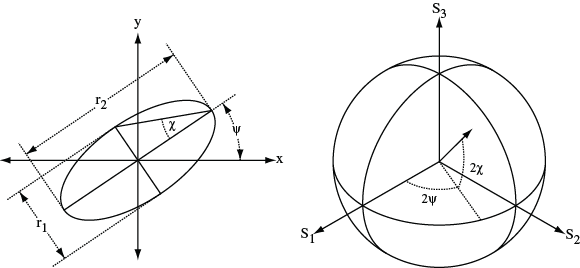Positive values of χ correspond to right-handed polarization states while negative values of χ are left-handed polarization states. χ = 0 corresponds to linear polarization while χ = π/4 is circularly polarized. On the Poincaré sphere, 2ψ and 2χ describe the longitude and lattitude of the principal eigenstate, respectively.

When specifying the eigenstates of the fiber, it is sufficient to give ψ and χ for one eigenstate because the second eigenstate is known to be orthogonal to the first.

Elliptical Basis Method

Any polarization state may be decomposed into a linear combination of the two orthogonal eigenstates of the fiber, which we label “a” and “b”. If ux and ux represent the two components of the electric field vector in the x-y basis (i.e., the Jones vector), then the corresponding components ua and ub can be calculated using the unitary transformation: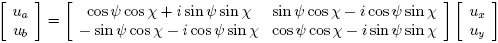where ψ and χ describe the principal eigenstate (“a”) of the fiber. In this new basis, the linear portion of the wave equations for ua and ub are decoupled. The linear portion of the propagation can therefore be performed separately on ua and ub in the spectral domain, using a technique analogous to that used in the scalar case.where ha and hb are given by: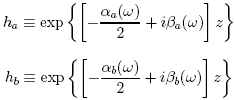When performing the nonlinear part of the propagation, the appropriate coupled nonlinear equations (with linear terms omitted) are [Menyuk, JQE 1989]: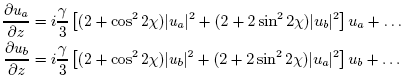where χ quantifies the degree of ellipticity of the eigenstates as described above. The (…) terms in the above expression denote additional nonlinear terms that average to zero when the birefringent beat length is much shorter than the nonlinear length. These additional terms are also identically zero when the eigenstates are circularly polarized.

After propagating through the desired number of steps, the final solution can be rotated from the elliptical basis (ua, ua) back into the Jones basis (ux,uy) by using the inverse transformation: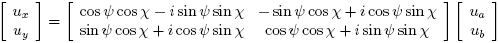Circular Basis Method

When the fiber birefringence is small, i.e., when the beat length is comparable to or larger than the nonlinear length, the additional terms in the nonlinear equations cannot be neglected. In this case, it is necessary to decompose the field into left- and right-hand circular polarization components before computing the nonlinear propagation. The circular components u+ and u can be computed from ux and uy using the following unitary transformation:With this transformation, the coupled nonlinear equations for u+ and u become (again omitting linear terms):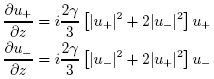where in this case no additional nonlinear terms have been neglected. Because the eigenstates of the fiber are not in general circularly polarized, the linear portion of the propagation is not as simple in the circular basis. After some algebra, one finds that the linear propagation can be be computed in the spectral domain, using the following matrix multiplication: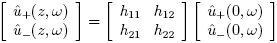where the matrix elements hnm are given by:and ha and hb are the same quantities given earlier in the context of the elliptical basis method.

After propagating through the desired number of steps, the final solution can be rotated from the circular basis (u+, u) back into the Jones basis (ux,uy) by using the inverse transformation: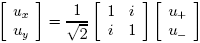The circular basis method is more accurate than the elliptical basis method because it does not neglect any nonlinear terms. The disadvantage of the circular method is that the stepsize dz must always be much smaller than the beat length in order to produce meaningful results. If the beat length is smaller than the nonlinear length, this requirement forces one to use a stepsize that is much smaller than the nonlinearity would otherwise dictate.

Syntax

A summary of the syntax and usage can be obtained from Matlab by typing “help sspropv” or “help sspropvc“.

The compiled mex file (sspropvc) can be invoked from Matlab using one of the following forms:

u1 = sspropvc(u0x,u0y,dt,dz,nz,alphaa,alphab,betapa,betapb,gamma);

u1 = sspropvc(u0x,u0y,dt,dz,nz,alphaa,alphab,betapa,betapb,gamma,psp,method);

u1 = sspropvc(u0x,u0y,dt,dz,nz,alphaa,alphab,betapa,betapb,gamma,psp,method,maxiter);

u1 = sspropvc(u0x,u0y,dt,dz,nz,alphaa,alphab,betapa,betapb,gamma,psp,method,maxiter,tol);

The last four arguments assume a default value if they are left unspecified. The corresponding Matlab m-file can be invoked using a similar syntax by replacing sspropvc with sspropv.

sspropvc may also be invoked with a single input argument, to specify options specific to the FFTW routines (discussed below):

sspropvc -option

Input Arguments

 u0x, u0y vector (N) Input optical field, specified by two length-N vector time sequences. u0x represents the x-component of the complex, slowly-varying envelope of the optical field, and u0y represents the corresponding y-component. The fields should be normalized so that |u0x|^2 + |u0y|^2 is the optical power. dt scalar The time increment between adjacent points in the vector u0. dz scalar The step-size to use for propagation nz scalar (int) The number of steps to take. The total distance propagated is therefore L = nz*dz alphaa, alphab scalar or vector (N) The linear power attenuation coefficients for the two eigenstates of the fiber. Here we use the labels “a” and “b” to denote the two eigenstates, which need not coincide with the x-y axes. Polarization dependent loss is modeled by using different numbers for alphaa and alphab.The loss coefficient may optionally be specified as a vector of the same length as u0x, in which case it will be treated as vector that describes a wavelength-dependent loss coefficient α(ω) in the frequency domain. (The function wspace.m in the tools subdirectory can be used to construct a vector with the corresponding frequencies.) betapa, betapb vector Real-valued vectors that specify the dispersion for each eigenstate (a, b) of the fiber. The dispersion can be specified to any polynomial order by using a betap vector of the appropriate length.Birefringence is accomodated by making the first elements betapa(1) and betapb(1) unequal. Differential group delay, or polarization mode dispersion is likewise treated by making the second elements betapa(2) and betapb(2) different. (See note below for a more complete discussion.)The propagation constant can also be specified directly by replacing the polynomial argument betap with a vector of the same length as u0x. In this case, the argument betap is treated as a vector describing propagation constant β(ω) in the frequency domain. (The function wspace.m in the tools subdirectory can be used to construct a vector with the corresponding frequencies.) gamma scalar A real number that describes the nonlinear coefficient of the fiber, which is related to the mode effective area and the nonlinear refractive index n2. psp scalar or vector (2) Principal eigenstate of the fiber, specified as a 2-vector containing the angles ψ and χ (see discussion above), psp = [ψ ,χ].If psp is a scalar, it is interpreted to be ψ, and χ is then taken to be zero. This corresponds to a linearly-birefringent fiber whose axes are oriented at an angle χ with respect to the x-y axes.If psp is left completely unspecified, it assumes a default value of [0,0], which means that the fiber eigenstates are linearly polarized along the x- and y- directions. method string String that specifies which method to use when performing the split-step calculations. The following methods are recognized “elliptical” or “circular”.When method = “elliptical”, sspropv will solve the equations by decomposing the input field into the (in general) elliptical eigenstates of the fiber.  This method is appropriate only in fibers where the birefringent beat length is much shorter than the nonlinear length.When method = “circular”, sspropv will instead solve the equations by decomposing the input field into a right- and left-circular basis. This method is more accurate, but requires that the step size be small compared to the beat length. maxiter scalar (int) The maximum number of iterations to make per step. If the solution does not converge to the desired tolerance within this number of iterations, a warning message will be generated. Usually this means that the chosen stepsize was too small. (default = 4) tol scalar Convergence tolerance: controls to what level the solution must converge when performing the symmetrized split-step iterations in each step. (default = 10–5.)

Output Arguments

 u1x, u1y vector (N) Output optical field, specified as two length-N vectors.

Options

Several internal options of the routine can be controlled by separately invoking sspropvc with a single argument:

sspropvc -savewisdom

sspropvc -forgetwisdom

The first command will save the accumulated FFTW wisdom to a file that can be later used. The second command causes sspropc to forget all of the accumulated wisdom. The last command forces FFTW to load the wisdom file from the current directory. The wisdom file (if it exists) is automatically loaded the first time sspropvc is executed. The name of the wisdom file is “fftw-wisdom.dat” for the double-precision version of the program and “fftwf-wisdom.dat” for the single-precision version. This can be changed by recompiling the code. The wisdom files can and are shared between the vector and scalar versions of SSPROP. Note that the wisdom files are platform- and machine-specific. You should not expect optimal performance if you use wisdom files that were generated on a different computer.

The following four commands can be used to designate the planner method used by the FFTW routines in subsequent calls to sspropc.

sspropvc -estimate

sspropvc -measure

sspropvc -patient

sspropvc -exhaustive

The default method is patient. These settings are reset when the function is cleared or when Matlab is restarted.

These options are only available in the compiled version of the routine.